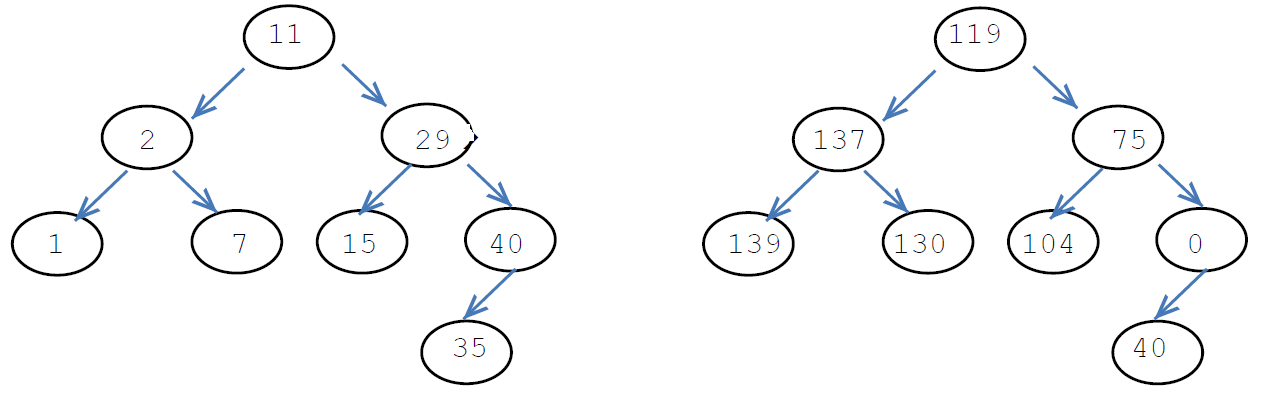New update is available. Click here to update.Close
Topic list
Convert Bst To The Greater Sum Tree
MEDIUM
30 mins11 upvotes
Trees
Binary Search Trees
Topics (Covered in this problem)
Problem solved
Skill meterTrees
-
-Binary Search Trees
-
-
Other topics
Problem solved
Skill meterStrings
-
-Matrices (2D Arrays)
-
--
-Sorting
-
-Binary Search
-
-Stacks & Queues
-
-Graph
-
-Dynamic Programming
-
-Greedy
-
-Tries
-
-Arrays
-
-SQL
-
-Heap
-
-Bit Manipulation
-
-
Solve problems & track your progress
Checkout your overall progress in every topic here
BecomeSensei
in DSA topics
Open the topic and solve more problems associated with it to improve your skills
Check out the skill meter for every topic
See how many problems you are left with to solve for cracking any stage. Score more than zero to get your progress counted.

# Convert Bst To The Greater Sum Tree

Medium0/80
Avg time to solve 30 mins
Success Rate 70 %Share11 upvotes

## Problem Statement

#### Note :

``````You need to modify the given tree only. You are not allowed to create a new tree.
``````
##### For example:
``````For the given binary search tree
````````````11 will be replaced by {15 + 29 + 35 + 40}, i.e. 119.
2 will be replaced by {7 + 11 + 15 + 29 + 35 + 40}, i.e. 137.
29 will be replaced by {35 + 40}, i.e. 75.
1 will be replaced by {2 + 7 + 11 + 15 + 29 + 35 + 40}, i.e. 139.
7 will be replaced by {11 + 15 + 29 + 35 + 40}, i.e. 130.
15 will be replaced by {15 + 29 + 35 + 40}, i.e. 104.
40 will be replaced by 0 {as there is no node with a value greater than 40}.
35 will be replaced by {40}, i.e. 40.
``````
Detailed explanation ( Input/output format, Notes, Constraints, Images )##### Sample Input 1:
``````2
5 2 7 1 3 6 8 -1 -1 -1 -1 -1 -1 -1 -1
4 2 5 -1 3 -1 6 -1 -1 -1 -1
``````
##### Sample Output 1:
``````21 29 8 31 26 15 0
11 18 6 15 0
``````
##### Explanation of Sample Input 1:
``````For the first test case, after converting the given binary tree into the greater sum tree, the level order traversal of the tree will be {21, 29, 8, 31, 26, 15, 0}, where 5 will be replaced by {6+7+8} i.e. 21, 3 will be replaced by {5+6+7+8} i.e. 26, 7 will be replaced by {8} i.e. 8, 1 will be replaced by {2+3+5+6+7+8} i.e. 33, 2 will be replaced by {3+5+6+7+8} i.e. 26, 6 will be replaced by {7+8} i.e. 15 and 8 will be replaced by 0 (because no node has a value greater than 8 in the given tree).

For the second test case, after converting the given binary tree into the greater sum tree, the level order traversal of the tree will be {11, 18, 6, 15, 0}, where, 4 will be replaced by {5+6} i.e. 11, 2 will be replaced by {3+4+5+6} i.e. 18, 5 will be replaced by {6} i.e. 6, 3 will be replaced by {4+5+6} i.e. 15 and 6 will be replaced by 0 (because no node has a value greater than 6 in the given tree).
``````
##### Sample Input 2:
``````2
11 2 29 1 7 15 40 -1 -1 -1 -1 -1 -1 35 -1 -1 -1
10 5 15 2 7 12 20 -1 -1 -1 -1 -1 -1 -1 -1
``````
##### Sample Output 2:
``````119 137 75 139 130 104 0 40
47 64 20 69 57 35 0
``````
##### Explanation of Sample Input 2:
``````For the first test case, after converting the given binary tree into the greater sum tree, the level order traversal of the tree will be {119, 137, 75. 130, 104, 0, 40}.

For the second test case, after converting the given binary tree into the greater sum tree, the level order traversal of the tree will be {47, 64, 20, 69, 57, 35, 0}.
``````AutoConsole